# Volume of Pyramid

##By VividMaths.com

Watch this video to discover how to use this formula to find the volume of a pyramid. An example involving a square pyramid is shown to aid your understanding.# Volume of a Pyramid, Deriving the Formula

##By mathematicsonline

complete explanation for volume of a pyramid here: http://pythagoreanmath.com/deriving-the-volume-of-a-pyramid/ Sum of integers squared video link:# How to Find the Volume of a Pyramid

##By Davitily

YouTube presents How to Find the Volume of a Pyramid an educational video resources on math.# The Inverted Pyramid News Article Style

##By expertvillage

Learn how to write in a journalistic style manner. All news articles are written in inverted pyramid style. An experts explains the history of inverted pyramid style and the how to write using this style# Relationships between sq pyramids and sq prisms part 2

##By TeacherTube Math

http://www.teachertube.com Using the relationships between square pyramids and square prisms to find a formula for volume of a square pyramid# Geometry - 19 - Pyramids - Volume, Lateral Area, and Total Area

##By thenewboston

This video shows how to find the volume, lateral area, and total surface area of a pyramid.# How to find the VOLUME of Cylinder, Cone, Cuboid, Prism, Pyramid for SSC CGL | Mensuration in HINDI

##By EduPoint

This Hindi video deals with, how to find the volume of Cylinder, Cone, Cuboid, Prism and Pyramid (triangular and square based). This mensuration video is very helpful for SSC CGL aspirants. volume of cylinder = π X(radius)^2 X height volume of cone = 1/3 X π X(radius)^2 X height volume of cuboid = length X breadth X height volume of prism = area of triangular base X height volume of pyramid = 1/3 X area of the base X height volume of cone = 1/3 X volume of cylinder of same base and height volume of pyramid (square base) = 1/3 X volume of cuboid of same base and height volume of pyramid (triangular base) = 1/3 X volume of prism of same base and height# 3D-Shapes: Vertices, Edges and Faces

##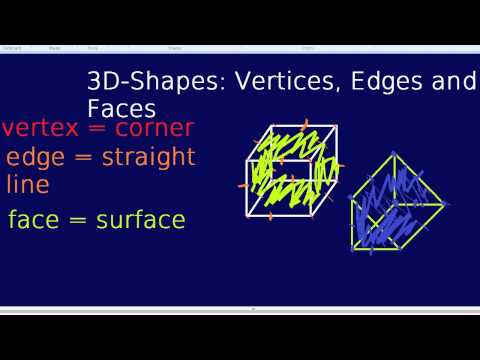By BespokeEducation

How to find edges and vertices. Spheres, triangular pyramids, square based pyramids, and an explanation of edges, vertices and faces.# Pyramids, prisms, cylinders and cones

##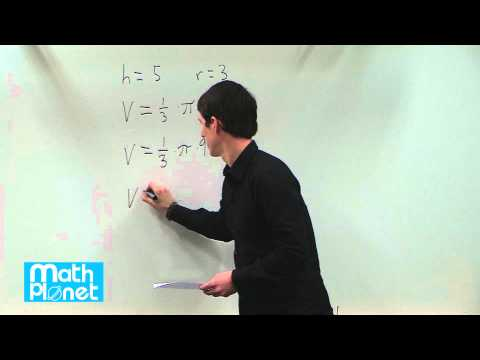By MathPlanetVideos

Find the volume of a cone with the height 5 and the radius 3.# Slicing 3D figures

##By Khan Academy

What happens when you slice vertically into a rectangular pyramid? What kind of geometric shape results?# The surface area and the volume of pyramids, prisms, cylinders and cones

##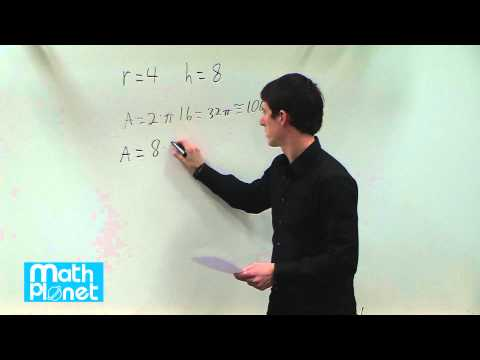By MathPlanetVideos

Find the surface area of a cylinder with the radius 4 and height 8# Geometry - Volume of Prisms, Cylinders, Pyramids and Cones: 7th grade math

##By Stan Lisle

In this lesson we show how to calculate the volume of several solid figures.# Volume word problems with cones, cylinders, and spheres

##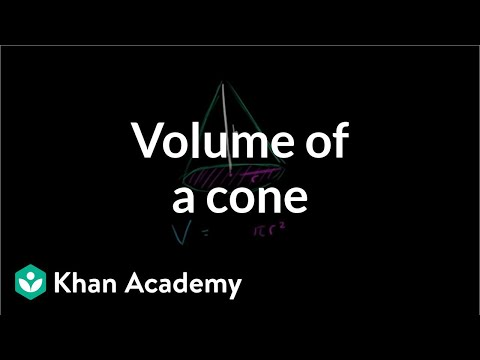By Khan Academy

Volume word problems with cones, cylinders, and spheres# Volume word problems with cones, cylinders, and spheres

##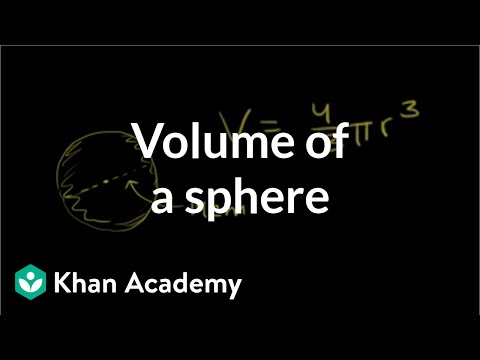By Khan Academy

Volume of a Sphere# Volume word problems with cones, cylinders, and spheres

##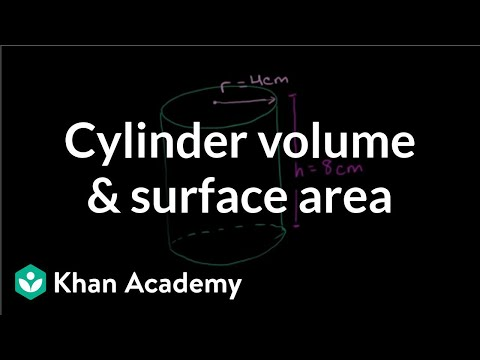By Khan Academy

Finding the volume and surface area of a cylinder# Surface and volume density word problems

##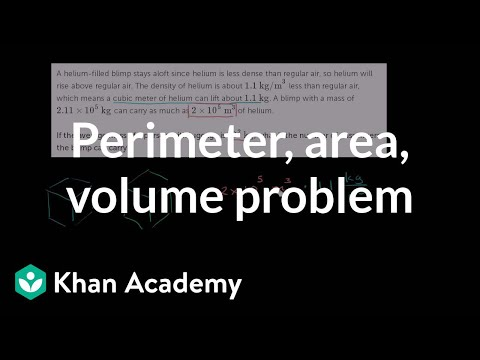By Khan Academy

Surface and volume density word problems# How Essay Style is Related to Essay Structure

##By The Critical Thinker Academy

In this video, the instructor emphasizes the difference between the structure and the style of an argumentative essay. He defines structure as those aspects of writing which a writer can outline or diagram. He shows graphics of an argumentative and an inverted pyramid structure. Style concerns what choices a writer makes to fit a certain task, purpose, and audience.# Quadrilateral overview | Perimeter, area, and volume | Geometry | Khan Academy

##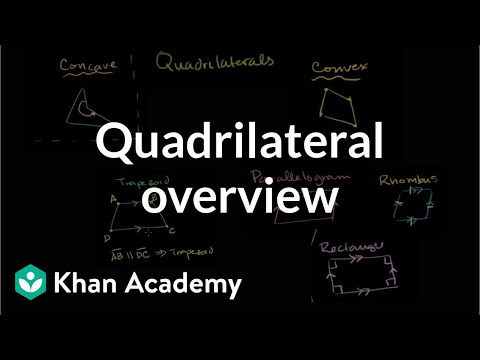By Khan Academy

Quad means four and lateral means line. A quadrilateral is literally a shape with four sides (lines) and four angles. Let's learn the difference between concave and convex quadrilaterals as well as trapezoids, parallelograms, and rhombi.# Quadrilateral properties | Perimeter, area, and volume | Geometry | Khan Academy

##By Khan Academy

How about this: we are given a 4-sided shape and asked to determine whether its properties qualify it to be called a quadrilateral (or category of quadrilaterals). Check it out.# Introducing Volume.

##By FutureSchoolVideos

This video is an introduction to understanding volume and standard units. The instructor uses animated blocks to help him describe volume.